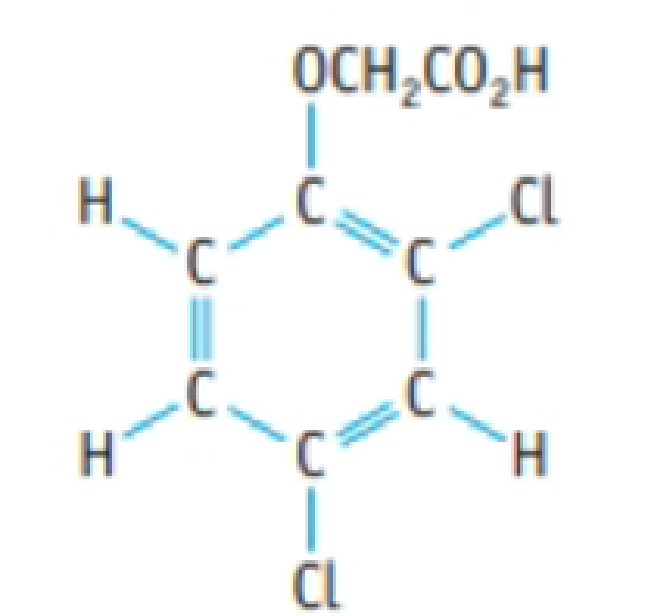# A herbicide contains 2,4-D (2,4-dichlorophenoxyacetic acid), C 8 H 6 Cl 2 O 3 . A 1.236-g sample of the herbicide was decomposed to liberate the chlorine as Cl − ion. This was precipitated as AgCl, with a mass of 0.1840 g. What is the mass percent of 2,4-D in the sample? 2,4-D (2,4-dichlorophenoxyacetic acid)### Chemistry & Chemical Reactivity

9th Edition
John C. Kotz + 3 others
Publisher: Cengage Learning
ISBN: 9781133949640

#### Solutions

Chapter
Section### Chemistry & Chemical Reactivity

9th Edition
John C. Kotz + 3 others
Publisher: Cengage Learning
ISBN: 9781133949640
Chapter 4, Problem 129IL
Textbook Problem
251 views

## A herbicide contains 2,4-D (2,4-dichlorophenoxyacetic acid), C8H6Cl2O3. A 1.236-g sample of the herbicide was decomposed to liberate the chlorine as Cl− ion. This was precipitated as AgCl, with a mass of 0.1840 g. What is the mass percent of 2,4-D in the sample?2,4-D (2,4-dichlorophenoxyacetic acid)

Interpretation Introduction

Interpretation:

The mass percentage of 2,4-D(2,4-dichlorophenoxyaceticacid),C8H6Cl2O3 in the given sample of compound has to be determined.

Concept introduction:

• For chemical reaction balanced chemical reaction equation written in accordance with the Law of conservation of mass.
• Law of conservation of mass states that for a reaction total mass of the reactant and product must be equal.
• The molar mass of an element or compound is the mass in grams of 1 mole of that substance, and it is expressed in the unit of grams per mol (g/mol).
• Mass percent of elements of a compound is the ratio of mass of element to the mass of whole compound and multiplied with hundred.
• Numberofmole=GivenmassofthesubstanceMolarmass

### Explanation of Solution

Here herbicide 2,4-D(2,4-dichlorophenoxyaceticacid) sample decomposed to liberate the chlorine as Cl ion. And it was precipitated as AgCl with a mass of 0.1840g

From the mass of  AgCl amount of 2,4-D(2,4-dichlorophenoxyaceticacid), and its mass can be calculated.

There is possibility to liberate 2molofCl from 1mol of C8H6Cl2O3.

Amount of AgCl produced in the reaction is,

0.1840g143.32g/mol=0.00128molAgCl

From this amount of AgCl the amount of C8H6Cl2O3 can be calculated as follows,

0

### Still sussing out bartleby?

Check out a sample textbook solution.

See a sample solution

#### The Solution to Your Study Problems

Bartleby provides explanations to thousands of textbook problems written by our experts, many with advanced degrees!

Get Started

Find more solutions based on key concepts
Each gene is a blueprint that directs the production of one or more of the body's organs. T F

Nutrition: Concepts and Controversies - Standalone book (MindTap Course List)

What gives amino acids their individual properties?

Biology: The Dynamic Science (MindTap Course List)

Define the following terms: a. chromosome b. chromatin

Human Heredity: Principles and Issues (MindTap Course List)

Name two strategies for decreasing a school-age childs sedentary behaviors.

Nutrition Through the Life Cycle (MindTap Course List)

A body cavity fully lined with tissue derived from mesoderm is called a(n) _____.

Biology: The Unity and Diversity of Life (MindTap Course List)

The expression F= ar + br22 gives the magnitude of the resistive force (in newtons) exerted on a sphere of radi...

Physics for Scientists and Engineers, Technology Update (No access codes included)

Can scientific inquiry probe further back in time than the big bang?

Oceanography: An Invitation To Marine Science, Loose-leaf Versin